Test: Real Analysis- 1

# Test: Real Analysis- 1

Test Description

## 20 Questions MCQ Test Topic-wise Tests & Solved Examples for IIT JAM Mathematics | Test: Real Analysis- 1

Test: Real Analysis- 1 for Mathematics 2022 is part of Topic-wise Tests & Solved Examples for IIT JAM Mathematics preparation. The Test: Real Analysis- 1 questions and answers have been prepared according to the Mathematics exam syllabus.The Test: Real Analysis- 1 MCQs are made for Mathematics 2022 Exam. Find important definitions, questions, notes, meanings, examples, exercises, MCQs and online tests for Test: Real Analysis- 1 below.
Solutions of Test: Real Analysis- 1 questions in English are available as part of our Topic-wise Tests & Solved Examples for IIT JAM Mathematics for Mathematics & Test: Real Analysis- 1 solutions in Hindi for Topic-wise Tests & Solved Examples for IIT JAM Mathematics course. Download more important topics, notes, lectures and mock test series for Mathematics Exam by signing up for free. Attempt Test: Real Analysis- 1 | 20 questions in 60 minutes | Mock test for Mathematics preparation | Free important questions MCQ to study Topic-wise Tests & Solved Examples for IIT JAM Mathematics for Mathematics Exam | Download free PDF with solutions
 1 Crore+ students have signed up on EduRev. Have you?
Test: Real Analysis- 1 - Question 1

### If A = {a, b, c} and R = {(a, a), (a, b) ( b, c), (b, b), (c, c), (c, a)} is a binary relation on A, then which one of the following is correct?

Detailed Solution for Test: Real Analysis- 1 - Question 1

Since, (a, a), (b, b), (c, c) ε R
Therefore, R is a reflexive relation
But, (a,b ) ε R and (b, a) R So, R is not a symmetric relation Also, (a, b), (b, cj 6 R.
Implies (a, c)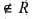Hence, R is not a transitive relation

Test: Real Analysis- 1 - Question 2

### If the cardinality of a set A is 4 and that of a set B is 3, then what is the cardinality of the set A Δ B?​

Detailed Solution for Test: Real Analysis- 1 - Question 2

Since, the sets A and B are not known thus cardinality of the set A Δ B cannot be determined.

Test: Real Analysis- 1 - Question 3

### Assertion (A): If events, A, B, C and D are mutually exhaustive, then (A ∪ B ∪ C)c = D. Reason (R): (A ∪ B ∪ C)c = D implies if any element is excluded from the sets A, B and C, then it is included in D.

Detailed Solution for Test: Real Analysis- 1 - Question 3

Both (A) and (R) are true and (R) is the correct explanation of (A).
Since, (A ∪ B ∪ C)c = Ac ∩ Bc ∩ Cc = D

Test: Real Analysis- 1 - Question 4

Elements of a population are classified according to the presence or absence of each of 3 attributes A, B and C. What is the number of smallest ultimate classes into which the population is divided?

Detailed Solution for Test: Real Analysis- 1 - Question 4

Elements of a population are classified according to the presence or absence of each of 3 attributes A, B and C. Then, the smallest number of smallest ultimate classes into which the population is divided, is 23 = 8

Test: Real Analysis- 1 - Question 5

If A and B are subsets of a set X, then what is {A ∩( X - B)} } ∪ B equal to?

Detailed Solution for Test: Real Analysis- 1 - Question 5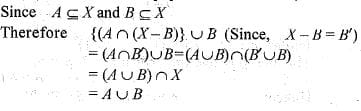Test: Real Analysis- 1 - Question 6

The total number of subsets of a finite set A has 56 more elements, then the total number of subsets of another finite set B. What is the number of elements in the set A?

Detailed Solution for Test: Real Analysis- 1 - Question 6

Let sets A and B have m and n elements, respectively,
Then 2m - 2n = 56 (According to question)
implies2n(2m-n - 1)
= 8 x 7 = (2)x 7 = 23(23- l )
On comparing implies n = 3
and m-n = 3
implies m = 6 and n = 3
Number of subsets of A = 23 + 56 = 64 = 26
Hence,Number of elements of in A = 6

Test: Real Analysis- 1 - Question 7

​If f(x) =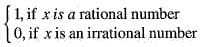Q. what is the value of (fof) (√3) ?

Detailed Solution for Test: Real Analysis- 1 - Question 7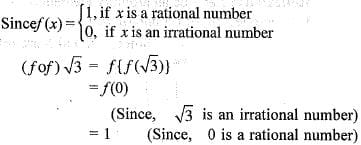Test: Real Analysis- 1 - Question 8

Consider the following statement.
I. Parallelism of lines is an equivalence relation.
II. xRy, if x is a father of y, is an equivalence relation. Which of the statements given above is/are correct?

Detailed Solution for Test: Real Analysis- 1 - Question 8

I. Let l, m, n are parallel lines and R is a relation.
Then l|| l, then R is reflexive,
and l || m and m || l, then R is symmetric.
Also, l || m, m || n => l | n, then R is transitive. Hence, R is an equivalence relation.
II. If x is father of y and y is not father of x then relation is not symmetric, thus relation is not equivalence

Test: Real Analysis- 1 - Question 9

The function f : R - > R defined by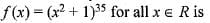Detailed Solution for Test: Real Analysis- 1 - Question 9

Since ,f(- 1) = f(1) = 235
i.e., Two real number 1 and -1 have the same image.
So, the function is not one-one and let y = (x2 + l )35 implies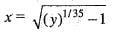Thus, every real number has no pre-image.
So, the function is not onto.
Hence, the function is neither one-one nor onto.

Test: Real Analysis- 1 - Question 10

If A and B are disjoint sets, then A ∩ (A' ∪ B) is equal to which one of the following?

Detailed Solution for Test: Real Analysis- 1 - Question 10

Since, A ∩ B = φ (given)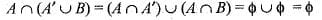Test: Real Analysis- 1 - Question 11

Let U= { 1 , 2 , 3 , ...,20}. Let A, B, C be the subsets of U.
Let A be the set of all numbers, which are prefect squares, B be the set of all numbers which are multiples of 5 and C be the set of all numbers, which are divisible by 2 and 3.
Q. Consider the following statements.
I. A, B, C are mutually exclusive.
II. A, B, C are mutually exhaustive.
III. The number of elements in the complement set of A∪ B is 12.
Q. Which of the statements given above the correct?

Detailed Solution for Test: Real Analysis- 1 - Question 11

U = { 1 ,2 ,3 , ...,20 } A = Set of all natural numbers which are perfect square
= {1,4 ,9 ,16 }
B = Set of all natural numbers which are multiple of 5
= {5,10,15,20}
C = Set of all natural numbers which are divisible by 2 and 3
= { 6 ,12 ,18 }
Since, A ∩ B ∩ C = φ
and A ∪ B = {1 ,4 ,9 ,1 6 ,5 ,10,15 ,20}
implies n ( A ∪ B ) = 8
n(A ∪ B)' = 20 - 8 = 12
hence A, B, C are mutually exclusive and the number of elements in the complement set of A ∪ B is 12 .

Test: Real Analysis- 1 - Question 12

The function f(x) = ex, x ε R is

Detailed Solution for Test: Real Analysis- 1 - Question 12

It is clear form the graph that f(x) = ex,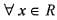is one- one but not onto. Since, range ≠ codomain, so f(x) is into.

Test: Real Analysis- 1 - Question 13

If A = P {1, 2} where P denotes the power set, then which one of the following is correct?

Detailed Solution for Test: Real Analysis- 1 - Question 13

A = P {1 ,2} = {φ, {1}, {2}, {1 ,2 }}
From above, it is clear that {1 , 2} ε A

Test: Real Analysis- 1 - Question 14

Let R and S be two equivalence relations on a set A.
Then,

Detailed Solution for Test: Real Analysis- 1 - Question 14

Given, R and S are relations on set A.
Then R ε A x A and S ≤ A x A
=> R ∩ C ≤ A x A
implies R ∩ S is also a relation on A.
Reflexivity: Let a be an arbitrary element of A. Then, a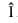A implies (a, a )R and (a, a)S,
[Since, R and S are reflexive]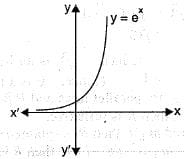implies(a, a) ε R ∩ S
Thus, (a , a ) ε R ∩ S for all a ε A.
So, R ∩ S is a reflexive relation on A.
Symmetry: Let a, b ε A such that (a, b) ε R ∩ S.
Then, (a, b) ε R ∩ S
implies (a, b) ε R and (a, b) ε S
implies (b, a) ε R and (b , a ) ε S
[Since R and S are symmetric]
implies (b, a) ε R ∩ S
Thus, (a, b) ε R ∩ S implies
(b, a) ε R ∩ S for all (a, b ) ε R ∩ S .
So, R ∩ S is symmetric on A.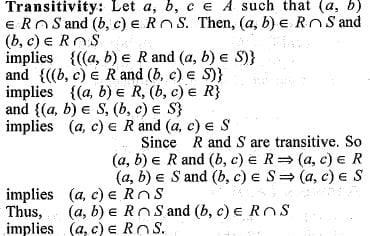So, R ∩ S is transitive on A.
Hence, R is an equivalence relation on A.

Test: Real Analysis- 1 - Question 15

If (1 + 3 + 5 + ...+ p )+ (1 + 3 + 5 + ...+ q) = ( l + 3 + 5 + ... + r) where each set of parentheses contains the sum of consecutive odd integers as shown, what is the smallest possible value o f (p + q + r) where p > 6?

Detailed Solution for Test: Real Analysis- 1 - Question 15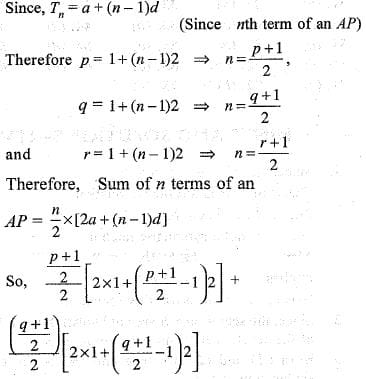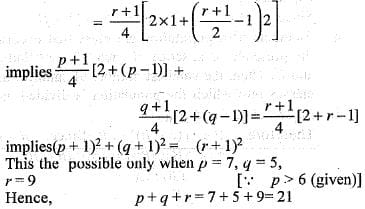Test: Real Analysis- 1 - Question 16

Let A = {x | x ≤ 9 , x ε N}. Let B = {a, b, c} be the subset of A where (a + b + c) is a multiple of 3. What is the largest possible number of subsets like B?

Detailed Solution for Test: Real Analysis- 1 - Question 16

Here,
A -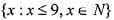= {1,2,3,4,5,6,7,8,9} ;
Total possible multiple of 3 are
= 3,6,9,12,15,18,21,24,27
But 3 and 27 are not possible.
6 → 1 + 2 + 3
9 → 2 + 3 + 4 , 5 + 3 + 1,6 + 2 + 1
12 → 9 + 2 + 1,8 + 3 + 1,7 + 1 + 4,
7 + 2 + 3 ,( 5+ 4 + 2 , 6 + 5 + 1, 5 + 4 + 3
15 → 9 + 4 + 2,9 + 5 + l, 8 + 6 + 1 , 8 + 5 + 2 , 8 + 4 + 3 , 7 + 6 + 2 , 7 + 5 +3 , 6 + 5 + 4
18 → 9 + 8+ 1,9 + 7 + 2,9 + 6 + 3,9 + 5 + 4, 8 + 7 + 3, 8 + 6 + 4,7 + 6 + 5
21→9 + 8 +4, 9 + 7 + 5, 8 + 7 + 6
24 → 9 + 8 + 7
Hence, total number of largest possible subsets are 30.

Test: Real Analysis- 1 - Question 17

A mapping f: R → R which is defined a s f( x ) = cos x; x ε R is

Detailed Solution for Test: Real Analysis- 1 - Question 17

Given , f(x) = cos x
It is clear from the figure that f(x) is neither one-one nor a onto function.
Since, whenever we drawn a line parallel to x-axis, then it intersects at infinite points to the curve. So, f(x) is not one-one.
And, range o f f ( x ) = [-1, 1]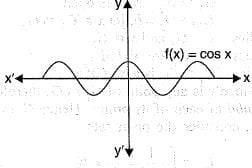Codomain of f(x) = R
Range of f(x) ≠ codomain of f(x)
Hence, f(x) is not onto.

Test: Real Analysis- 1 - Question 18

If n(A) = 115, n{B) = 326, n(A — B) = 47, then what is n(A ∪ B) equal to?

Detailed Solution for Test: Real Analysis- 1 - Question 18

Now,
n(A -B )= n(A) - n(A ∩ B)
implies 47 = 115 - n(A ∩ B)
or n(A ∩ B) = 68
n(A ∪ B) = n(A) + n(B) — n(A ∩ B)
= 115 + 326 - 68 = 373

Test: Real Analysis- 1 - Question 19

In a town of 10000 families it was found that 40% family buy newspaper A, 20% buy newspaper B and 10% families buy newspaper C, 5% families buy A and B, 3% buy B and C and 4% buy A and C. If 2% families buy all the three newspaper, then number of families which buy A only is

Detailed Solution for Test: Real Analysis- 1 - Question 19

Here.
n(A)= 40% of 10000 = 4000
n(B)= 20% of 10000 = 2000
n(C)= 10% of 10000 =1000
n(A ∩ B) = 5% of 10000 = 500
n(B ∩ C) = 3% of 10000 = 300
n(C ∩ A) = 4% of 10000 = 400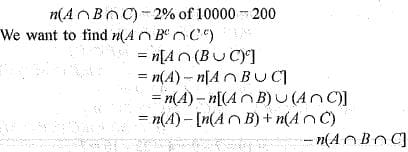= 4000 - [500 + 400 - 200]
= 4000- 700 - 3300

Test: Real Analysis- 1 - Question 20

A survey shows that 63% of the Americans like cheese whereas 76% like apples. If x% of the Americans like both cheese and apples, then

Detailed Solution for Test: Real Analysis- 1 - Question 20

Let A denote the set ot Americans who like cheese and let B denote the set of Americans who like apples.
Let population of American be 100.
Then, n(A) = 63, n(B) =76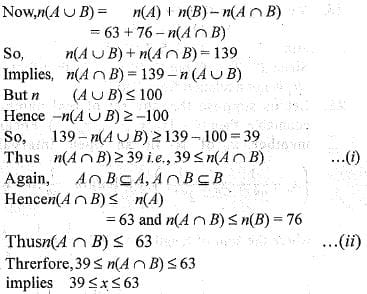## Topic-wise Tests & Solved Examples for IIT JAM Mathematics

27 docs|150 tests
 Use Code STAYHOME200 and get INR 200 additional OFF Use Coupon Code
Information about Test: Real Analysis- 1 Page
In this test you can find the Exam questions for Test: Real Analysis- 1 solved & explained in the simplest way possible. Besides giving Questions and answers for Test: Real Analysis- 1, EduRev gives you an ample number of Online tests for practice

## Topic-wise Tests & Solved Examples for IIT JAM Mathematics

27 docs|150 tests